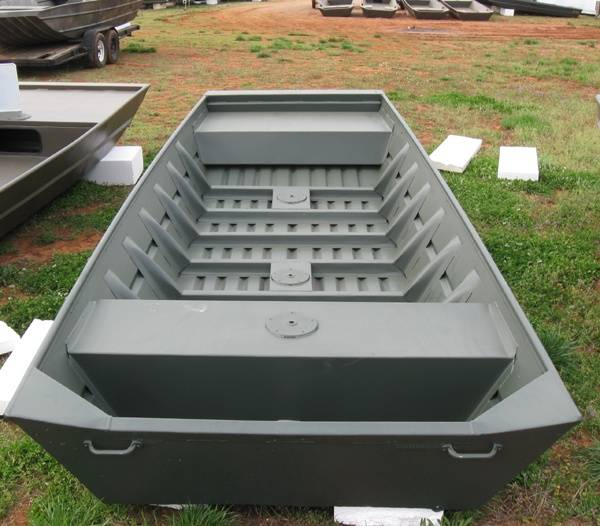# Archimedes principle and shapes of the body

Archimedes principle of the force of upthrust = weight of water displaced by the body immersed , but is this law independent of the shape of this body. If we use the idea that the upthrust = P*A on the lower side - P*A on the upper side , if we use it on a rectangle or a square , the only change here is the pressure. But what if we used a body like trapezium (where the area also changes) , the smaller base is facing down, i made some calculations using Pressure * area to figure out if it will float or sink , and theoretically it will sink , i meant to do this experiment in real life , but couldn't find any trapeziums. So can anybody help me here? will it float? will it sink? and why (specially if the answer is that it will float )?

I dont see how its possible to have any shape who's cross section when viewed from the top is different than its cross section when viewed from the bottom.

Your comment about a trapezium implies that there would be no water pressure on the angled sides? Of course that is not the case.

SteamKing
Staff Emeritus
Homework Helper
Any floating body can be overloaded, even the rectangular one.

I dont see how its possible to have any shape who's cross section when viewed from the top is different than its cross section when viewed from the bottom.

Your comment about a trapezium implies that there would be no water pressure on the angled sides? Of course that is not the case.

Correct me if i am wrong ( i am new to the world of physics) , but regarding the angled sides, doesn't pascal's second law apply here? if it does the force should be perpendicular to the surface , no upwards. If it doesn't apply , can you tell me please to calculate the water pressure on those angled sides ? PS: i am not arguing if the thing will float or sink, i know it will float , just trying to know why , thanks

Chestermiller
Mentor
The pressure acts perpendicular to the angled sides, and you need to look at the components of these pressure forces in the vertical direction (integrated over the area of the angled sides). When you integrate the vertical components of the pressure over the portion of the body that is submerged, you find that the net vertical force on the body is exactly equal to the weight of volume of water displaced.

•1 person
D H
Staff Emeritus
The pressure acts perpendicular to the angled sides, and you need to look at the components of these pressure forces in the vertical direction (integrated over the area of the angled sides). When you integrate the vertical components of the pressure over the portion of the body that is submerged, you find that the net vertical force on the body is exactly equal to the weight of volume of water displaced.
Mathematically, ##\vec F = - \oint_S P(S)\,\hat n(S)\,dS##, where ##P(S)## is the pressure at some point on the surface and ##\hat n(S)## is the unit outward normal to the surface at that point. This is a mess in general. However, the divergence theorem tells us how to rewrite this surface integral as a volume integral. This volume integral is much more tractable than is the surface integral and it is this volume integral that leads to Archimedes' principle.

•1 person
Chestermiller
Mentor
Mathematically, ##\vec F = - \oint_S P(S)\,\hat n(S)\,dS##, where ##P(S)## is the pressure at some point on the surface and ##\hat n(S)## is the unit outward normal to the surface at that point. This is a mess in general. However, the divergence theorem tells us how to rewrite this surface integral as a volume integral. This volume integral is much more tractable than is the surface integral and it is this volume integral that leads to Archimedes' principle.
Yes. I had this approach in mind. In my response, I just wanted to explain how the pressure on the slanted sides of the body comes into play. This is a point of confusion for many new initiates.

Chet

SteamKing
Staff Emeritus
Homework Helper
I wonder if this boat is like the 'trapeziums' the OP couldn't find.

It's wider at the top than the bottom.•1 person
Thanks guys , you really helped me